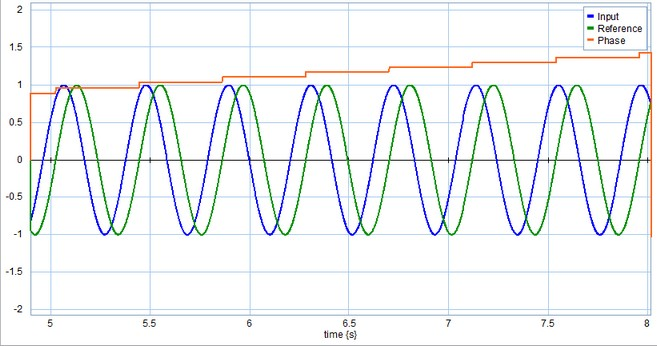﻿ 20-sim webhelp > Library > Signal > Signal Processing > PhaseSensor

# PhaseSensor

## Library

Signal\Signal Processing

## Use

Domains: Continuous. Size: 1-D. Allowed in: Block Diagrams.

## Description

This model measures the phase of a harmonic signals (i.e. periodic signals, that go through zero twice every period) with respect to another harmonic signal. Two inputs are used:

 • Reference
 • Input

The PhaseSensor block measures the zero crossings of both the input and the reference, to assess the phase difference between both. The phase output is the phase of the input signal with respect to the reference signal. The measurement will not work if the input sines have a non-zero

mean.## Interface

 Inputs Description input the signal of which the phase is measured reference with respect to this signal Outputs output the measured phase Parameters initial Initial output value allow_negative_phase True: measured phase between -pi and +pi False: measured phase between 0 and 2*pi